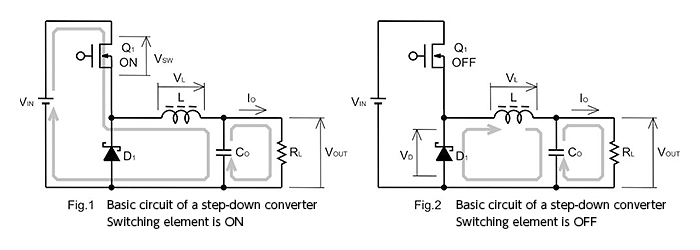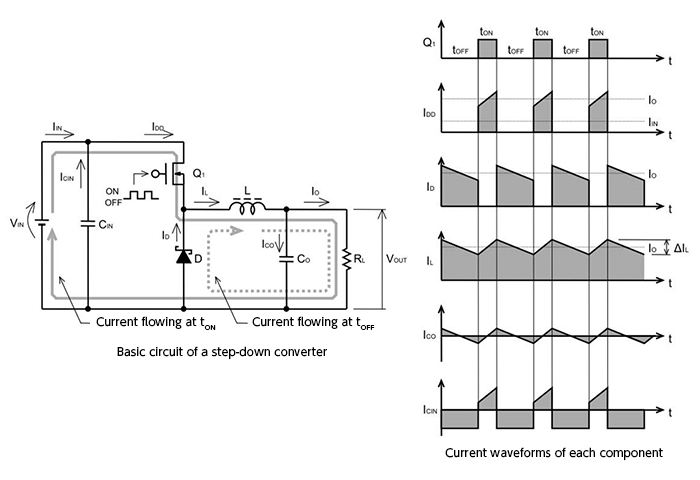Technical Information Site of Power Supply Design

• Basic Knowledge
• DC/DC
• Selection of Inductors and Capacitors for DC/DC Converters
• Basic Operation of Step-Down Converters

2017.04.27 DC/DC

# Basic Operation of Step-Down Converters

Selection of Inductors and Capacitors for DC/DC Converters

In this chapter, we explain the methods for selecting the inductors and capacitors that are important components of step-down DC/DC converters in particular, the selection of which can have a major impact on performance and characteristics. In order to understand the components, it is necessary to know about the basic operation of a step-down DC/DC converter and the flow of currents in its operation. Hence by way of a review, we begin by explaining the basic operation and current paths.

Basic operation of step-down converters

Below, the basic circuit and operation of a step-down converter, as well as the flow of current, are illustrated.• Fig. 1 shows the state in which the switching element Q1 is turned on.
• When Q1 is turned on, current passes from the input pin VIN to the coil L to charge the output smoothing capacitor CO, and the output current IO is supplied.
• The current flowing in the coil L at this time generates a magnetic field, and electrical energy is converted into magnetic energy and accumulated.
• Fig. 2 illustrates the state in which the switching element Q1 is turned off.
• When Q1 is turned off, the diode D1 turns on, and energy accumulated in L is discharged to the output.

The basic circuit diagram shown is of a diode-rectifier type (non-synchronous rectification) step-down circuit. In the case of synchronous rectification, D1 is replaced with a switching element (transistor), which is turned on and off in operation opposite that of Q1, but the basics are the same.

The diagrams below summarize Fig. 1 and Fig. 2 above, showing the voltage or current waveform at each major node.It is easy to see how, as Q1 is turned on and off, the drain current ID, inductor current IL, as well as the output capacitor current ICO and input capacitor current ICIN change.

With a firm understanding of the circuit operation, one can understand the jobs performed by the various components and the characteristics these components should have.

Hereafter, with this operation and current flows in mind, we will proceed to explain inductor selection.

#### Key Points:

・In DC/DC converter design, selection of inductors and input and output capacitors is particularly important.

・Circuit operation, current paths, and the roles played by the different components must be understood.